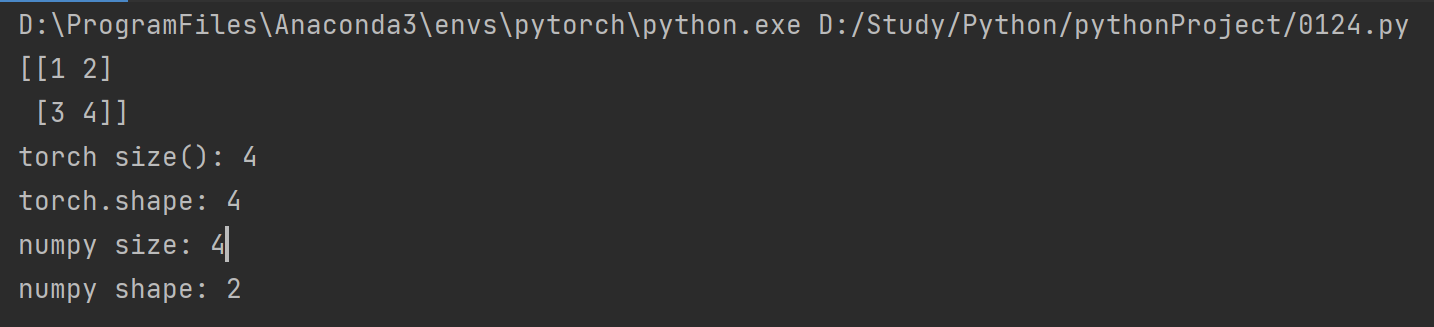有别于numpy中size的用法（用来计算数组和矩阵中所有元素的个数），pytorch的size具有和shape一样计算矩阵维度大小的作用。

``````import torch
import numpy as np

torch.manual_seed(1)
a=torch.randn(3,4)
b=np.arange(1,5)
b=b.reshape([2,2])
# print(a)
print(b)
print("torch size():",a.size(1))
print("torch.shape:",a.shape)
print("numpy size:",b.size)
print("numpy shape:",b.shape)
``````